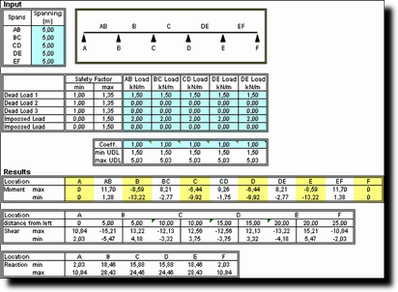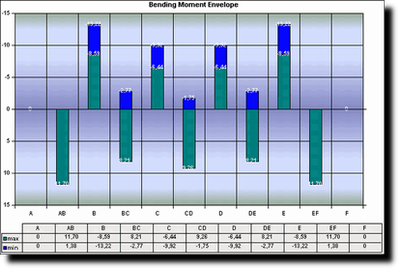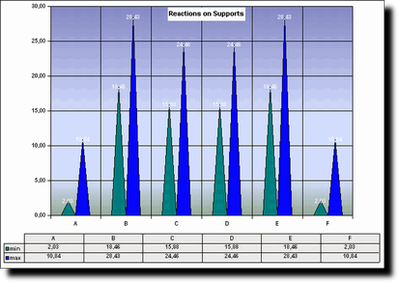.

# Continuous Beam

Continuous Beam is composed by a set of independent Excel spreadsheets, each capable to analyse a different beam type.

Continuous Beam can only be used for calculating the bending moment and shear force diagram of a multispanning continuous beam, loaded with universal distributed load. Calculations are carried out automatically using the Moment Redistribution Method. Results are printed in numerical tables and colour graphs.

All the required information can be inputted in the sky-blue cells of the Data sheet. Calculations are carried out in three separate sheets (one for each major type of loading combinations) and exported as a numerical table at the bottom of the sheet.